geometric proofs of trigonometric identitiesIntroduction
Below, some proofs are presented of trigonometric identities.
The proofs do not use any trigonometric formula or rule, what makes them quite special.

Identity Nr. 1
arctan( 1 2
) + + arctan( 1 3
) = arctan(1 )
Proof A.
See figure 1. below.
Pictured are 4 squares.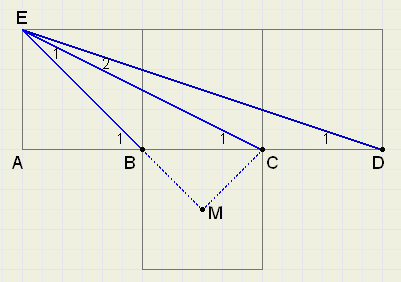fig.1

Since:
LC1 = arctan( 1 2
)
LD1 = arctan( 1 3
)
LB1 = arctan(1 )
We must prove:
LC1 + LD1 = LB1.........1)
DEMC ~ DDAE, because:
1. LA = LM = 900
2. MC = 3.ME
so:
LE1 = LD1
which changes ........1) into:
LC1 + LE1 = LB1
This is a basic geometric theorem.
(remember: 1800 - LB1 = 1800 - (LC1+LE1)
This concludes proof A.

Proof B.
See figure 2. below: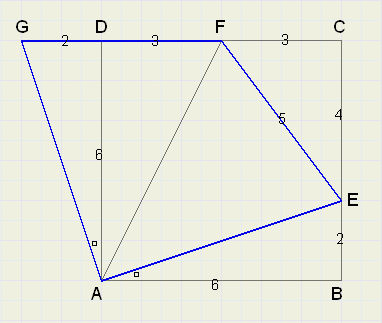fig.2

Note: EF = 5, because of theorem of Pythagoras.

DADG is the rotation of DABE over 900= LGAE.

Now observe polygon AEFG.
FG = FE
AG = AE
so:
DAFG and DAFE are congruent
so:
LGAF = LFAE = 450
arctan
æ 1 3
ö
­­
èø
+  arctan
æ 1 2
ö
­­
èø
=  arctan 1
Which concludes proof B.

Identity Nr. 2
sin(200 ) + sin(400 ) = sin(800 )
Proof.
See figure 3. below: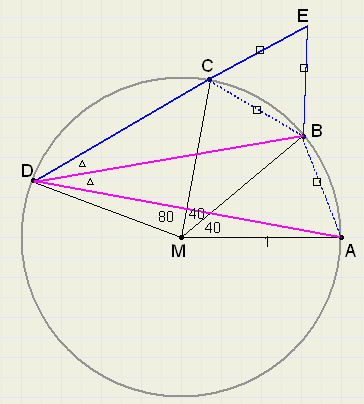fig.3

M is the center of a circle with radius = 1.
Points A,B,C,D are located on the circle at the indicated angles.

Chord DC is extended by CE = BC.
Now DCBE is equilateral because:
LDCB = 0.5(3600 - 1200) = 1200 .........(see appendix 1.)
LECB = 600 ....so
LCBE = LCEB = 600....so
BE = BC = AB
and we see:
DDBE is congruent to DDBA....so
DE = DA ............and since
CE + DC = DE
2sin(200 )+ 2sin(400 ) = 2sin(800 )........(see appendix 2)
sin(200 )+ sin(400 ) = sin(800 )
and we have proved the 2nd identity.

Appendix 1 (measuring angles by arcs)
See figure 4. below: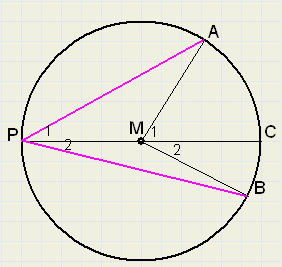fig.4

By:...... arc AB = 800 we mean : LAMB = 800

Since
LM1 = LA + LP1.......exterior angle of DPMA
MA = MP
LP1= LA
LM1 = 2*LP1
LP1 = 0.5*LM1 = 0.5* arc AC.....2
.....1 and .....2 combined:
LP1,2 = 0.5*LM1,2 = 0.5*arc AB
In words:
an angle located on a circle is half the size of the associated arc.

Appendix 2 (sine and chord relation)
See figure 5. below: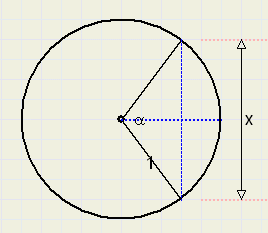fig.5
sin
æ a 2
ö
­­
èø
=  x 2

x = 2 ·  sin
æ a 2
ö
­­
èø